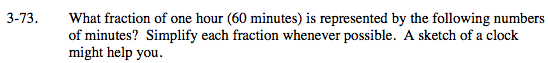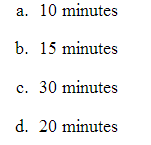### Home > MC2 > Chapter 3 > Lesson 3.2.2 > Problem3-73

3-73.Consider each number of minutes as a fraction of one hour or 60 minutes. Simplify each fraction by dividing both the numerator and denominator by a common factor.

$\frac{10}{60} = \frac{1}{6}$

$\frac{30}{60} = \frac{1}{2}$

Explore the problem with the eTool below.
Click the link at right for the full version of the eTool: MC2 3-73 HW eTool